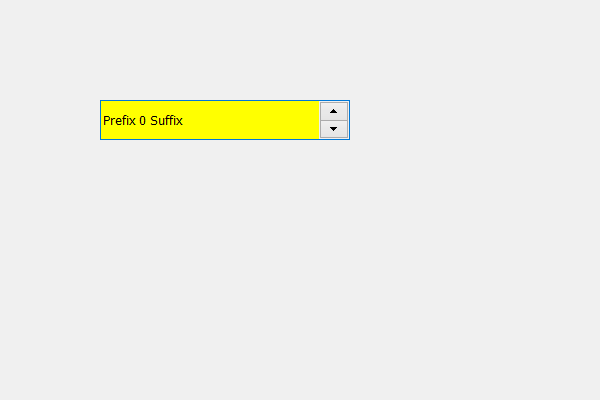Related Articles

# PyQt5 QSpinBox – Adding background color to the line edit part

• Last Updated : 17 May, 2020

In this article we will see how we can set background color to the line edit part of the spin box, line edit part is the text part of the spin box where we can see the text and input the user value.

In order to do this we have to do the following –
1. Create a spin box
2. Get the spin box line edit object
3. Add order to the line edit object

Syntax :

```# get the line edit object
line = self.spin_box.lineEdit()

# setting background color to the line edit object
line.setStyleSheet("QLineEdit"
"{"
"background-color : yellow;"
"}")
```

Below is the implementation

 `# importing libraries``from` `PyQt5.QtWidgets ``import` `*` `from` `PyQt5 ``import` `QtCore, QtGui``from` `PyQt5.QtGui ``import` `*` `from` `PyQt5.QtCore ``import` `*` `import` `sys`` ` ` ` `class` `Window(QMainWindow):`` ` `    ``def` `__init__(``self``):``        ``super``().__init__()`` ` `        ``# setting title``        ``self``.setWindowTitle(``"Python "``)`` ` `        ``# setting geometry``        ``self``.setGeometry(``100``, ``100``, ``600``, ``400``)`` ` `        ``# calling method``        ``self``.UiComponents()`` ` `        ``# showing all the widgets``        ``self``.show()`` ` `    ``# method for widgets``    ``def` `UiComponents(``self``):``        ``# creating spin box``        ``self``.spin ``=` `QSpinBox(``self``)`` ` `        ``# setting geometry to spin box``        ``self``.spin.setGeometry(``100``, ``100``, ``250``, ``40``)`` ` `        ``# setting prefix to spin``        ``self``.spin.setPrefix(``"Prefix "``)`` ` `        ``# setting suffix to spin``        ``self``.spin.setSuffix(``" Suffix"``)`` ` `        ``# get the line edit object``        ``line ``=` `self``.spin.lineEdit()`` ` `        ``# setting background color to the line edit object``        ``line.setStyleSheet(``"QLineEdit"``                           ``"{"``                           ``"background-color : yellow;"``                           ``"}"``)`` ` ` ` ` ` `# create pyqt5 app``App ``=` `QApplication(sys.argv)`` ` `# create the instance of our Window``window ``=` `Window()`` ` `# start the app``sys.exit(App.``exec``())`

Output :Attention geek! Strengthen your foundations with the Python Programming Foundation Course and learn the basics.

To begin with, your interview preparations Enhance your Data Structures concepts with the Python DS Course. And to begin with your Machine Learning Journey, join the Machine Learning – Basic Level Course

My Personal Notes arrow_drop_up